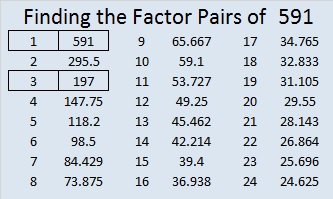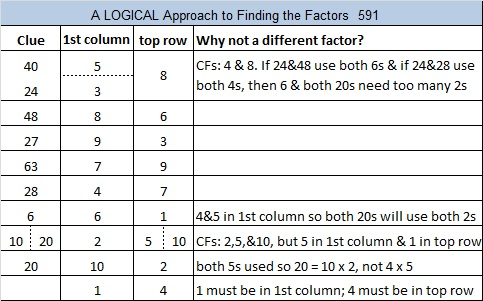# 591 and a Sometimes Impossible Level 6

5 + 9 + 1 = 15, a multiple of 3 so 591 is divisible by 3.

However, since 9 is a multiple of 3, it isn’t necessary to include it in the equation, so I simple say 5 + 1 = 6, a multiple of 3 so 591 is divisible by 3.

591 is the hypotenuse of the Pythagorean triple 84-585-591. Which of 591’s factors is the greatest common factor of those three numbers?

—————————————————————————————————

I love that Joseph Nebus wrote that the FIND THE FACTORS puzzles are “a fun recreational mathematics puzzle even if the level 6’s and sometimes level 5’s will sometimes feel impossible.”

Judge for yourself. Is this Level 6 puzzle impossible or not!Print the puzzles or type the solution on this excel file: 10 Factors 2015-08-17

—————————————————————————————————

• 591 is a composite number.
• Prime factorization: 591 = 3 x 197
• The exponents in the prime factorization are 1 and 1. Adding one to each and multiplying we get (1 + 1)(1 + 1) = 2 x 2 = 4. Therefore 591 has exactly 4 factors.
• Factors of 591: 1, 3, 197, 591
• Factor pairs: 591 = 1 x 591 or 3 x 197
• 591 has no square factors that allow its square root to be simplified. √591 ≈ 24.31049—————————————————————————————————